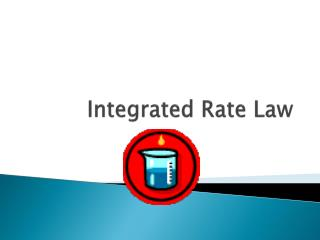DownloadDownload PresentationIntegrated Rate Law

# Integrated Rate Law

Download Presentation## Integrated Rate Law

- - - - - - - - - - - - - - - - - - - - - - - - - - - E N D - - - - - - - - - - - - - - - - - - - - - - - - - - -
##### Presentation Transcript

1. Integrated Rate Law

2. Rate laws can be converted into equations that tell us what the concentration of the reactants or products are at any time • Calculus required to derive the equations but not to use them

3. Zero Order • Rate = k • With calculus it can be changed to an equation that relates the starting conc ([A]o) to the conc at any other time (t) • [A] = -kt + [A]o

4. Graph • Plot of [A] vs t is linear with a slope equal to k

5. Uses of Equation • Find the conc of a reactant at some time after the reaction started • Find the time required for a given fraction of a sample to react • Find the time required for a reactant to reach a certain concentration

6. First Order Reactions • Rate = k[A] • ln[A] = - kt + ln[A]o

7. Graphs • Plot of ln[A] vs t is linear

8. Example • The first order constant for the hydrolysis of a certain insecticide in water at 12°C is 1.45/yr. A quantity of this insecticide is washed into a lake in June, leading to an overall concentration of 5 x 10-7 g/cm3 of water. Assume that the effective temp of the lake is also 12°C. A) what is the conc of the insecticide in June of the following year? B) How long will it take for the conc of the insecticide to drop to 3.0 x 10-7 g/cm3 ?

9. Example • The decomposition of dinitrogen pentoxide is studied over time and the results are given in in the table on pg.573. a) Verify if this is a first order reaction. b) Calculate the value of the rate constant c) Find the concentration after 150 sec.

10. Half Life • Time required for the concentration of a reactant to decrease to halfway between its initial and final values • Time when [A]= ½ [A]o • t1/2 = .693/k

11. Example • What is the half life of the insecticide in the lake from the previous example?

12. Second Order Reactions • Rate = k[A]2 • 1/[A] = kt + 1/[A]o • Plot of 1/[A] vs t is linear

13. Graph • Plot of 1/[A] vs t is linear

14. Example • The following data was obtained for the decomposition of nitrogen dioxide. Is the reaction first or second order? What is the rate constant? Time (s) [NO2] 0 .0100 50 .0079 100 .0065 200 .0048 300 .0038